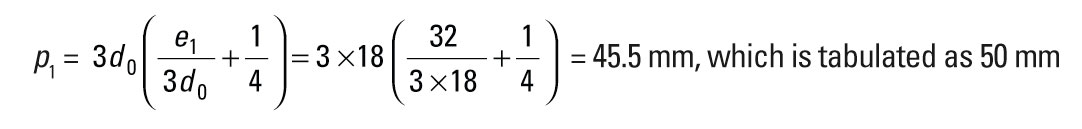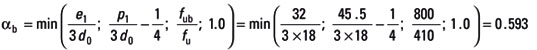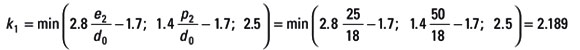# AD 348: Bolt resistance tables in the Eurocode Blue Book

This AD Note provides clarification of resistance values given in SCI publication P363 Steel building design: Design data (the Eurocode Blue Book).

The publication covers Class 4.6, 8.8 and 10.9 bolts, including non-preloaded and preloaded hexagon head bolts and countersunk bolts. Resistances for preloaded bolts include values for serviceability limit state and ultimate limit state. The calculation of resistances is described in the explanatory notes, Section 11.1 of the publication.

For Class 4.6 non-preloaded bolts, two tables of resistances are provided; for Class 8.8 and 10.9 non-preloaded bolts, three tables are provided.

For each bolt class, the first table gives tension resistance, shear resistance and minimum thickness to avoid punching shear, all determined in accordance with Table 3.4 of BS EN 1993-1-8. Although the punching shear resistance needs to be verified, this is not a common mode of failure in practical situations. The publication provides the minimum thickness required to ensure punching shear does not occur. Note that the dimensions of preloaded and non-preloaded bolts are different and therefore, for the same bolt size, the minimum thickness to prevent punching shear differs for the two types of bolts.

The second table gives bearing resistances for various ply thicknesses. The bearing resistance depends on the bolt arrangement, defined by e1, e2, p1 and p2. The end distance e1 has been taken as twice the bolt diameter and resistances have been calculated for these values of e1. However, the values of e1 printed in the tables have been rounded up to the nearest 5 mm. The edge distance e2 has been chosen to match common practice; values rounded to 5 mm have been chosen. The values of pitch, p1 and p2, have been chosen such that resistance values based on them are not more critical than those based on e1 (the ‘exact’ value rather than the rounded value) and e2, and they also have been rounded up to the nearest 5 mm. Examples of values in the second table are illustrated as follows:

Page C-303, Table 2, Class 8.8, M16 bolts:

e2 = 25 mm

e1 = 2d = 2×16 = 32 mm, which is tabulated as 35 mmp2 = 2e2 = 2 × 25 = 50 mm

For this bolt arrangement:For a ply thickness of 10 mm in S275:This is the value tabulated in P363.

If the resistance calculation were carried out using the tabulated values of e1 = 35 mm and p1 = 50 mm, then the resistance would increase to 74.4 kN.

The third table, for bolt classes 8.8 and 10.9, gives bearing resistances for increased values of e1, e2, p1 and p2, which give higher bearing resistance. As for the second table, values have been chosen according to an exact multiple of bolt or hole diameter and then rounded up to the nearest 5 mm. Resistances have been calculated based on the exact values, not the rounded values. The calculation has been based on the following values
of e1, e2, p1 and p2:

e2 = 1.5d0

e1 = 3d

p1 = 3.75d0

p2 = 3d0

The third table is not given for class 4.6 bolts because increasing the bolt spacing only has a modest effect. For an increased bearing resistance, a higher bolt class is recommended.

Contact: Edurne Nunez Moreno
Tel: 01344 636525